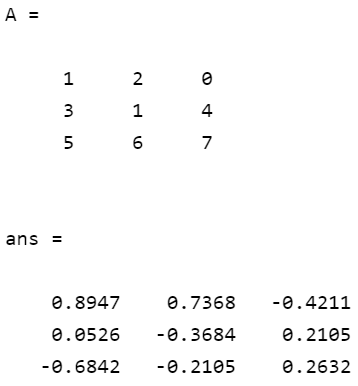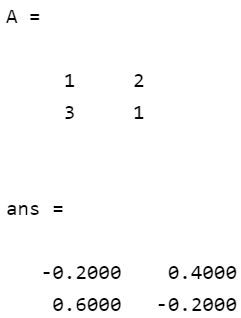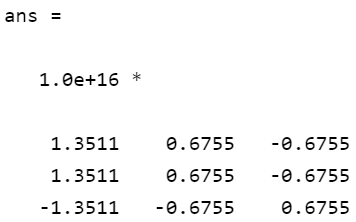Related Articles

# Find inverse of matrix in MATLAB

• Last Updated : 28 Apr, 2021

Inverse function in MATLAB is used to find the inverse of a matrix. Suppose A is a matrix and B is the inverse of a then A*B will be an identity matrix. This function computes the inverse of a square matrix. This is used while solving linear equations. We can compute the inverse of a matrix by passing it to inv().

Syntax:

inv(A)

Parameters:

It takes a matrix as parameter.

Returns:

It returns a matrix which is inverse of input matrix.

Below are some examples which depict how to compute the inverse of a matrix in MATLAB.

Example 1: This example takes a 3×3 matrix as input and computes its inverse using inv() function.

## Matlab

 `% Defining matrix``A = [1 2 0; 3 1 4; 5 6 7]`` ` `% Getting inverse matrix``inv(A)`

Output:Example 2: Here is another example that takes a 2×2 matrix as input and computes its inverse.

## Matlab

 `% Defining matrix``A = [1 2; 3 1]`` ` `% Getting inverse matrix``inv(A)`

Output:Example 3: This example uses a singular matrix and tries to find its inverse. It will show a warning that the matrix is a singular matrix. Different versions of MATLAB gave a different value of inverse for singular matrix. This is due to the different versions of Math Kernel Library used in different versions of MATLAB.

## Matlab

 `% Defining matrix``A = [2 4 6;2 0 2;6 8 14]`` ` `% Getting inverse matrix``inv(A)`

Output:

`warning: matrix singular to machine precision, rcond = 1.34572e-17`My Personal Notes arrow_drop_up